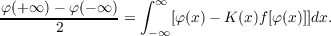#### Vol. 20, No. 1, 1967

 Download this articleFor screen For printingRecent Issues Vol. 325: 1  2 Vol. 324: 1  2 Vol. 323: 1  2 Vol. 322: 1  2 Vol. 321: 1  2 Vol. 320: 1  2 Vol. 319: 1  2 Vol. 318: 1  2Online Archive Volume: Issue:The Journal Subscriptions Editorial Board Officers Contacts Submission Guidelines Submission Form Policies for Authors ISSN: 1945-5844 (e-only) ISSN: 0030-8730 (print) Special Issues Author Index To Appear Other MSP Journals
On the equation φ(x) = xx+1K(ξ)f[φ(ξ)]

### Morton Lincoln Slater

Vol. 20 (1967), No. 1, 155–166
##### Abstract

Suppose K(x) measurable and 0 < K(x) 1 for x (−∞,). Suppose f(u) convex for u [0,1],f(0) = 0,f(u) > 0 for u (0,1), and f(u) = 1 f(1)(1 u) + O(1 u)1+δ as u 1 for some δ > 0. (Example: f(u) = up,p 1.) Theorem: The equation ()φ(x) = xx+1K(ξ)f[φ(ξ)] has a solution φ(x) satisfying 0 < φ(x) 1 for x (−∞,) if and only if eαx[1 K(x)]dx < where α is the largest real root of α = f(1)(1 eα). Furthermore, if φ is any such solution of (), then the limits φ(±∞) exist and satisfyPrimary: 45.30
##### Milestones